# ShellSort

• Difficulty Level : Medium
• Last Updated : 17 Aug, 2021

ShellSort is mainly a variation of Insertion Sort. In insertion sort, we move elements only one position ahead. When an element has to be moved far ahead, many movements are involved. The idea of shellSort is to allow exchange of far items. In shellSort, we make the array h-sorted for a large value of h. We keep reducing the value of h until it becomes 1. An array is said to be h-sorted if all sublists of every h’th element is sorted.

Following is the implementation of ShellSort.

Attention reader! Don’t stop learning now. Get hold of all the important DSA concepts with the DSA Self Paced Course at a student-friendly price and become industry ready.  To complete your preparation from learning a language to DS Algo and many more,  please refer Complete Interview Preparation Course.

In case you wish to attend live classes with experts, please refer DSA Live Classes for Working Professionals and Competitive Programming Live for Students.

## C++

 `// C++ implementation of Shell Sort``#include  ``using` `namespace` `std;` `/* function to sort arr using shellSort */``int` `shellSort(``int` `arr[], ``int` `n)``{``    ``// Start with a big gap, then reduce the gap``    ``for` `(``int` `gap = n/2; gap > 0; gap /= 2)``    ``{``        ``// Do a gapped insertion sort for this gap size.``        ``// The first gap elements a[0..gap-1] are already in gapped order``        ``// keep adding one more element until the entire array is``        ``// gap sorted``        ``for` `(``int` `i = gap; i < n; i += 1)``        ``{``            ``// add a[i] to the elements that have been gap sorted``            ``// save a[i] in temp and make a hole at position i``            ``int` `temp = arr[i];` `            ``// shift earlier gap-sorted elements up until the correct``            ``// location for a[i] is found``            ``int` `j;           ``            ``for` `(j = i; j >= gap && arr[j - gap] > temp; j -= gap)``                ``arr[j] = arr[j - gap];``            ` `            ``//  put temp (the original a[i]) in its correct location``            ``arr[j] = temp;``        ``}``    ``}``    ``return` `0;``}` `void` `printArray(``int` `arr[], ``int` `n)``{``    ``for` `(``int` `i=0; i

## Java

 `// Java implementation of ShellSort``class` `ShellSort``{``    ``/* An utility function to print array of size n*/``    ``static` `void` `printArray(``int` `arr[])``    ``{``        ``int` `n = arr.length;``        ``for` `(``int` `i=``0``; i ``0``; gap /= ``2``)``        ``{``            ``// Do a gapped insertion sort for this gap size.``            ``// The first gap elements a[0..gap-1] are already``            ``// in gapped order keep adding one more element``            ``// until the entire array is gap sorted``            ``for` `(``int` `i = gap; i < n; i += ``1``)``            ``{``                ``// add a[i] to the elements that have been gap``                ``// sorted save a[i] in temp and make a hole at``                ``// position i``                ``int` `temp = arr[i];` `                ``// shift earlier gap-sorted elements up until``                ``// the correct location for a[i] is found``                ``int` `j;``                ``for` `(j = i; j >= gap && arr[j - gap] > temp; j -= gap)``                    ``arr[j] = arr[j - gap];` `                ``// put temp (the original a[i]) in its correct``                ``// location``                ``arr[j] = temp;``            ``}``        ``}``        ``return` `0``;``    ``}` `    ``// Driver method``    ``public` `static` `void` `main(String args[])``    ``{``        ``int` `arr[] = {``12``, ``34``, ``54``, ``2``, ``3``};``        ``System.out.println(``"Array before sorting"``);``        ``printArray(arr);` `        ``ShellSort ob = ``new` `ShellSort();``        ``ob.sort(arr);` `        ``System.out.println(``"Array after sorting"``);``        ``printArray(arr);``    ``}``}``/*This code is contributed by Rajat Mishra */`

## Python3

 `# Python3 program for implementation of Shell Sort` `def` `shellSort(arr):``    ``gap ``=` `len``(arr) ``/``/` `2` `# initialize the gap` `    ``while` `gap > ``0``:``        ``i ``=` `0``        ``j ``=` `gap``        ` `        ``# check the array in from left to right``        ``# till the last possible index of j``        ``while` `j < ``len``(arr):``    ` `            ``if` `arr[i] >arr[j]:``                ``arr[i],arr[j] ``=` `arr[j],arr[i]``            ` `            ``i ``+``=` `1``            ``j ``+``=` `1``        ` `            ``# now, we look back from ith index to the left``            ``# we swap the values which are not in the right order.``            ``k ``=` `i``            ``while` `k ``-` `gap > ``-``1``:` `                ``if` `arr[k ``-` `gap] > arr[k]:``                    ``arr[k``-``gap],arr[k] ``=` `arr[k],arr[k``-``gap]``                ``k ``-``=` `1` `        ``gap ``/``/``=` `2`  `# driver to check the code``arr2 ``=` `[``12``, ``34``, ``54``, ``2``, ``3``]``print``(``"input array:"``,arr2)` `shellSort(arr2)``print``(``"sorted array"``,arr2)` `# This code is contributed by Shubham Prashar (SirPrashar)`

## C#

 `// C# implementation of ShellSort``using` `System;` `class` `ShellSort``{``    ``/* An utility function to``       ``print array of size n*/``    ``static` `void` `printArray(``int` `[]arr)``    ``{``        ``int` `n = arr.Length;``        ``for` `(``int` `i=0; i 0; gap /= 2)``        ``{``            ``// Do a gapped insertion sort for this gap size.``            ``// The first gap elements a[0..gap-1] are already``            ``// in gapped order keep adding one more element``            ``// until the entire array is gap sorted``            ``for` `(``int` `i = gap; i < n; i += 1)``            ``{``                ``// add a[i] to the elements that have``                ``// been gap sorted save a[i] in temp and``                ``// make a hole at position i``                ``int` `temp = arr[i];` `                ``// shift earlier gap-sorted elements up until``                ``// the correct location for a[i] is found``                ``int` `j;``                ``for` `(j = i; j >= gap && arr[j - gap] > temp; j -= gap)``                    ``arr[j] = arr[j - gap];` `                ``// put temp (the original a[i])``                ``// in its correct location``                ``arr[j] = temp;``            ``}``        ``}``        ``return` `0;``    ``}` `    ``// Driver method``    ``public` `static` `void` `Main()``    ``{``        ``int` `[]arr = {12, 34, 54, 2, 3};``        ``Console.Write(``"Array before sorting :\n"``);``        ``printArray(arr);` `        ``ShellSort ob = ``new` `ShellSort();``        ``ob.sort(arr);` `        ``Console.Write(``"Array after sorting :\n"``);``        ``printArray(arr);``    ``}``}` `// This code is contributed by nitin mittal.`

## Javascript

 ``

Output:

```Array before sorting:
12 34 54 2 3
Array after sorting:
2 3 12 34 54
```

Time Complexity: Time complexity of above implementation of shellsort is O(n2). In the above implementation gap is reduce by half in every iteration. There are many other ways to reduce gap which lead to better time complexity. See this for more details.

Snapshots: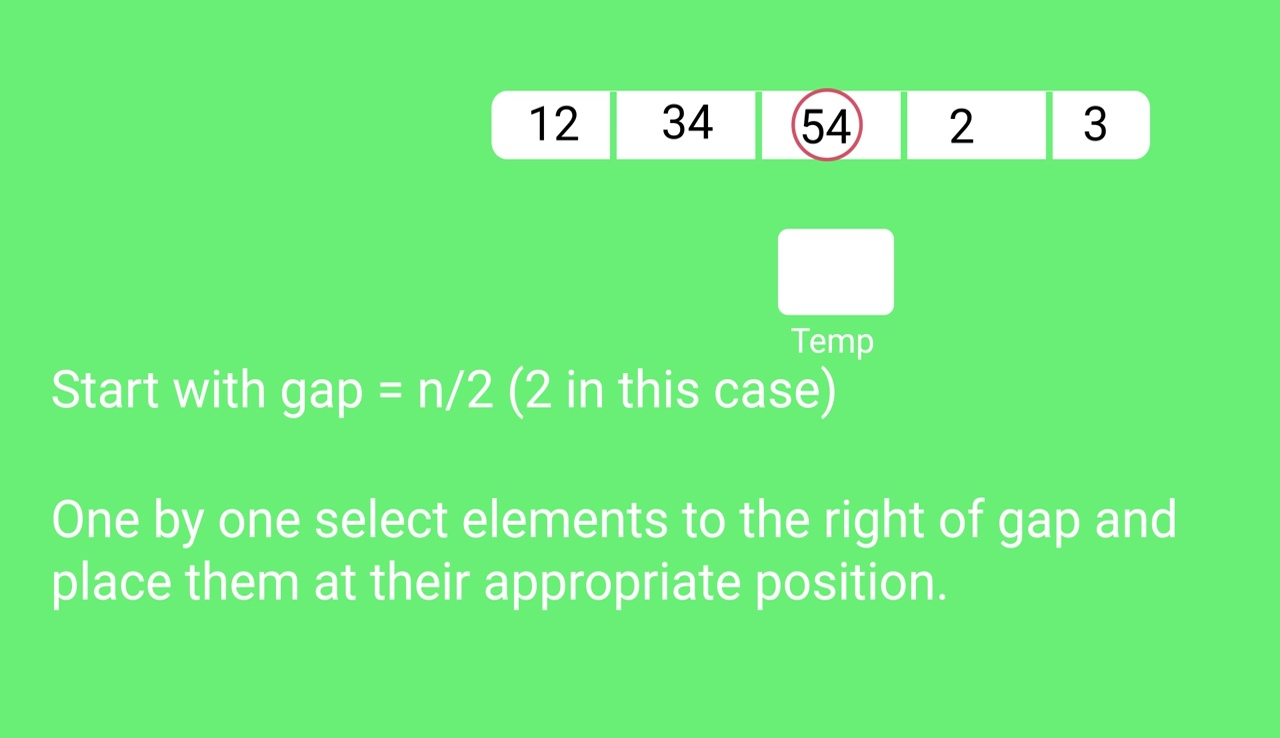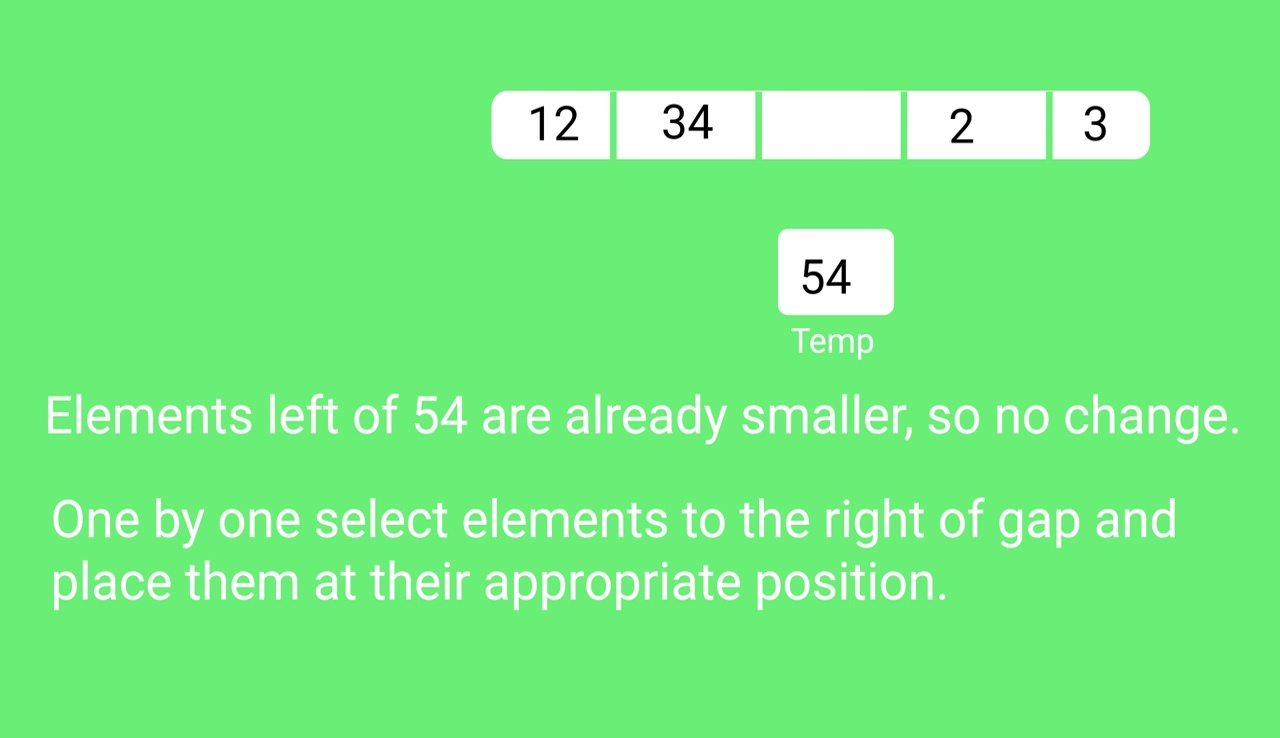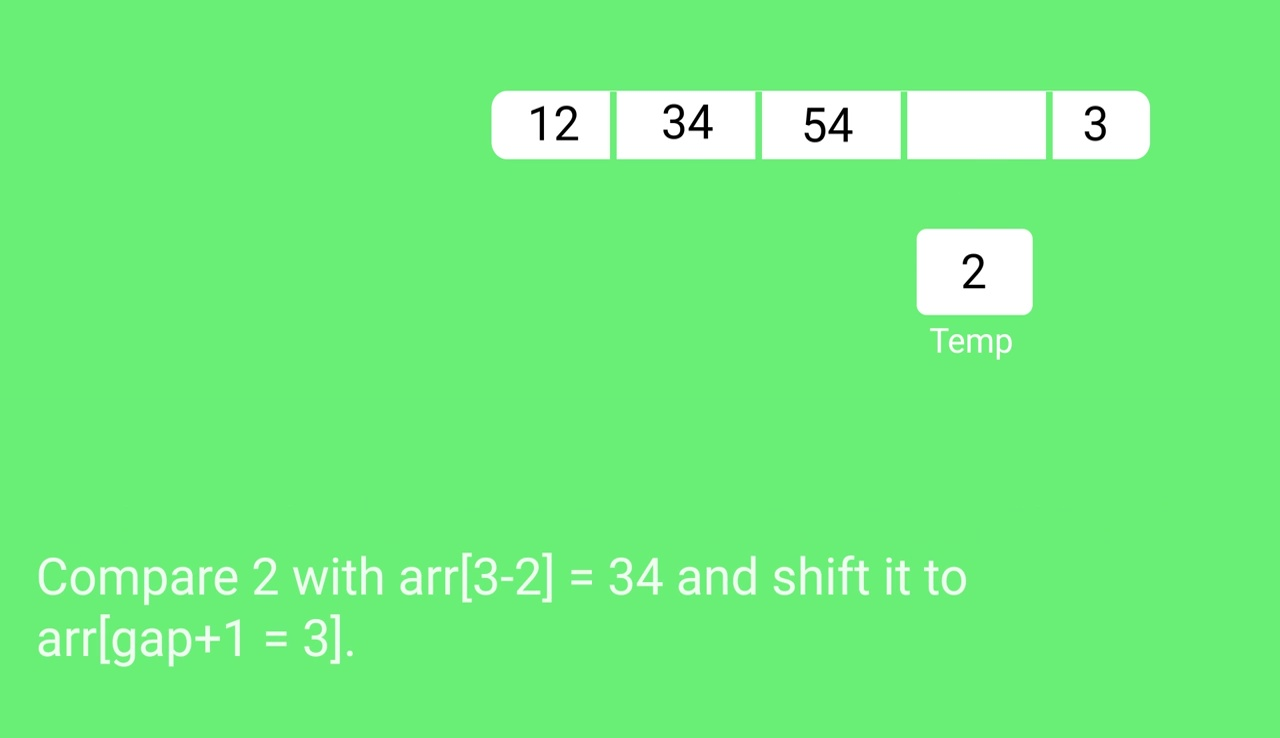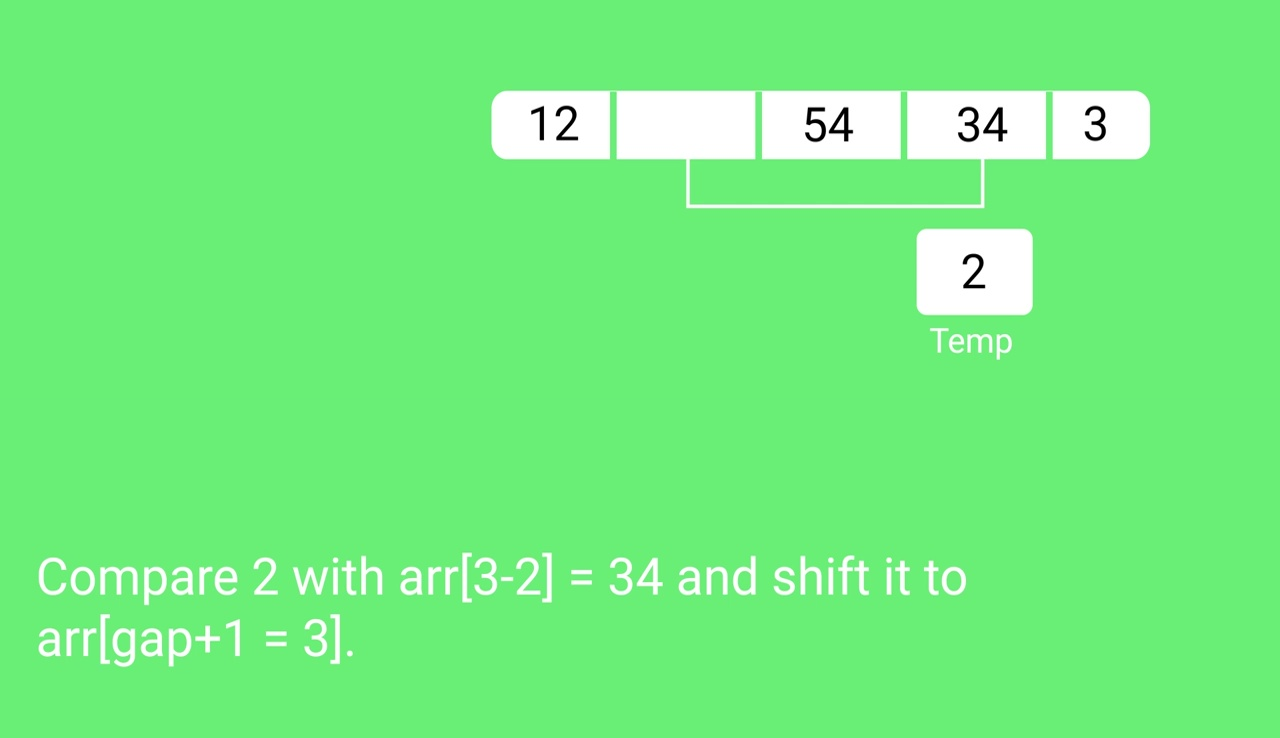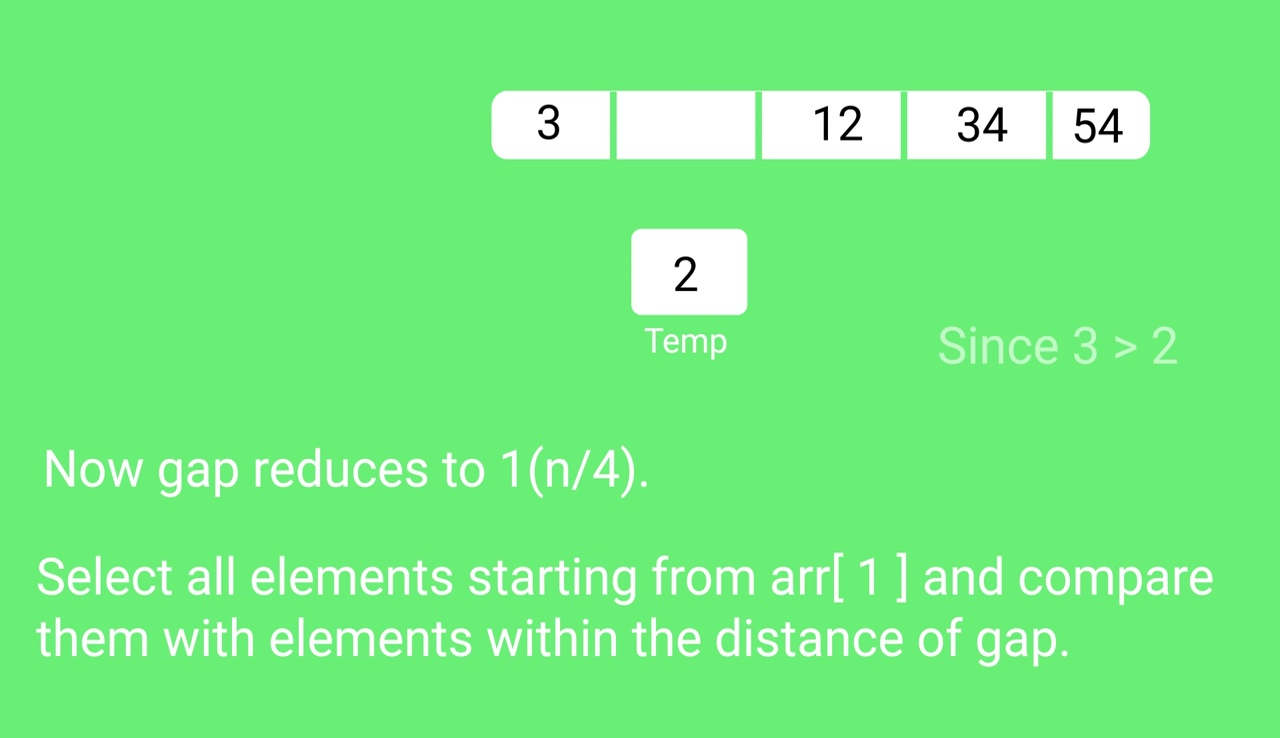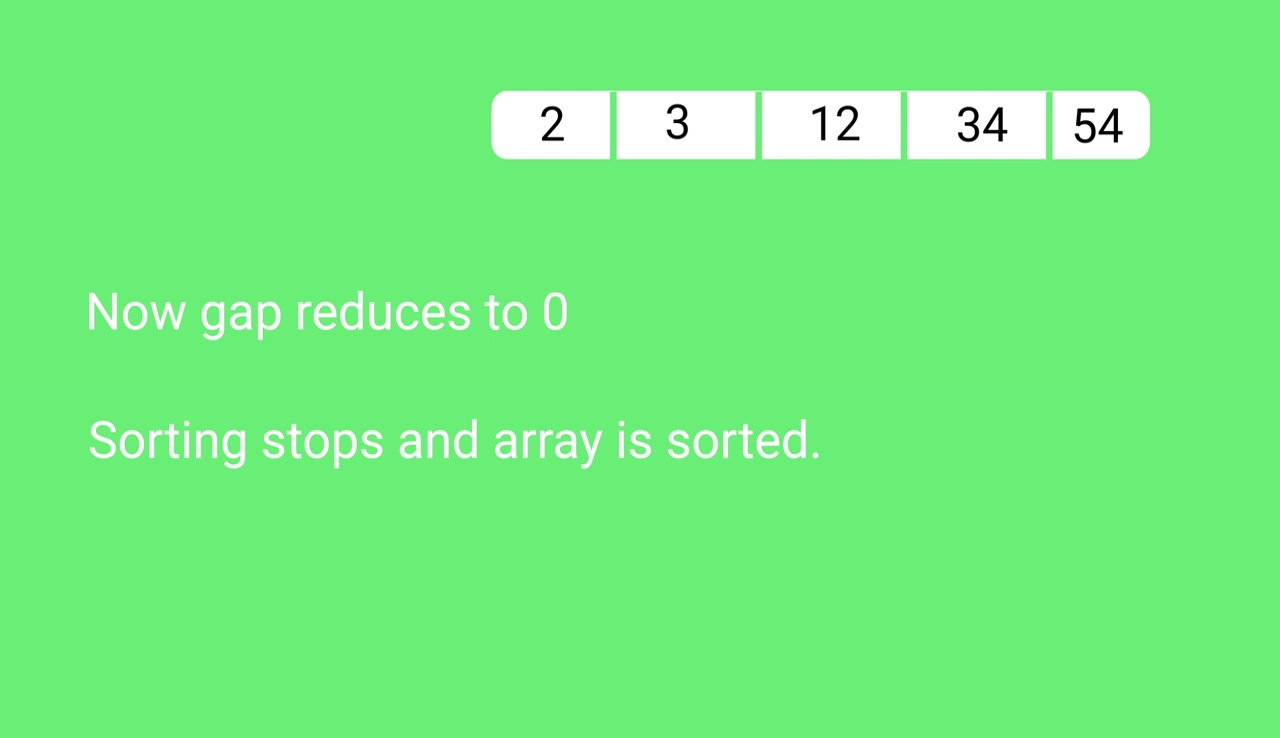## Quiz on Shell Sort

Other Sorting Algorithms on GeeksforGeeks/GeeksQuiz:

My Personal Notes arrow_drop_up